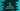# C++ program to find the sum of two numbers using friend function## How to add two times using a friend function in C++:

In this post, we will learn how to add two numbers using a friend function. You will learn what is a friend function, how to write a friend function and how to use it to find the sum of two numbers in C++.

Let’s learn first what is a friend function and why it is used.

### Friend function:

In object-oriented programming, we can’t access the private and protected members of a class without creating an object of that class. Only derived classes can access the protected members of a class.

There is another way to access the private and protected members of a class, and this can be done by using a friend function.

A friend function is declared in the body of a class and friend keyword is used for friend functions.

### Example of friend function in C++:

Let me show you a simple example of friend function.

``````#include <iostream>
using namespace std;

class Message
{
private:
string message;
friend void printMessage();
void Print()
{
cout << message << endl;
}
};

void printMessage()
{
Message m;
m.message = "Hello";
m.Print();
}

int main()
{
printMessage();
}``````

In this example,

• Message class has one private string variable message, one friend function printMessage and one private function Print. The Print function prints the value of message.
• The printMessage function is defined outside the class and it is called from the main() function.
• Even though message and Print() are private in the Message class, you can see that these values can be accessed from the printMessage function.

If you run this program, it will print the below result:

``Hello``

### Example of friend function to add two numbers:

Let’s use a friend function to add two numbers:

``````#include <iostream>
using namespace std;

class Calculator
{
private:
int first, second;
friend int addNumbers(Calculator, int i, int j);
{
return first + second;
}
};

int addNumbers(Calculator c, int firstNum, int secondNum)
{
c.first = firstNum;
c.second = secondNum;

}

int main()
{
Calculator c;
int firstNum, secondNum;

cout << "Enter the first number: " << endl;
cin >> firstNum;

cout << "Enter the second number: " << endl;
cin >> secondNum;

cout << "Sum: " << addNumbers(c, firstNum, secondNum) << endl;
}``````
• Calculator is a class with two private integer variables first and second.
• The addNumbers is a friend function that returns the sum of first and second.
• The user input numbers are stored in the firstNum and secondNum variables. It calls the addNumbers function to calculate the sum of firstNum and secondNum. It also passes an instance of Calculator to the friend function.
• In addNumbers function, we can access the private variables first and second as this is a friend function. It assigns firstNum to first and secondNum to second and calls Add() to find the sum.

If you run this program, it will print the output as below: